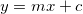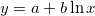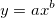Never Pet Marmots

npm

regression2.0.1 • Public • Published

Installation

This module works on node and in the browser. It is available as the 'regression' package on npm. It is also available on a CDN.

npm

npm install --save regression

Usage

Data is passed into the model as an array. A second parameter can be used to configure the model. The configuration parameter is optional. null values are ignored. The precision option will set the number of significant figures the output is rounded to.

Configuration options

Below are the default values for the configuration parameter.

Properties

• equation: an array containing the coefficients of the equation
• string: A string representation of the equation
• points: an array containing the predicted data in the domain of the input
• r2: the coefficient of determination (R2)
• predict(x): This function will return the predicted value

API

regression.linear(data[, options])

Fits the input data to a straight line with the equation. It returns the coefficients in the form [m, c].

regression.exponential(data[, options])

Fits the input data to a exponential curve with the equation. It returns the coefficients in the form [a, b].

regression.logarithmic(data[, options])

Fits the input data to a logarithmic curve with the equation. It returns the coefficients in the form [a, b].

regression.power(data[, options])

Fits the input data to a power law curve with the equation. It returns the coefficients in the form [a, b].

regression.polynomial(data[, options])

Fits the input data to a polynomial curve with the equation. It returns the coefficients in the form [an..., a1, a0]. The order can be configure with the order option.

Development

• Install the dependencies with npm install
• To build the assets in the dist directory, use npm run build
• You can run the tests with: npm run test.

Keywords

Install

npm i regression